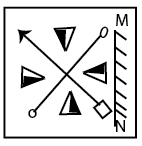Question 199

# From the answer figures, find out the figure which is the exact mirror image of thequestion figure, when the mirror is placed on the line MN.Solution

The arrow in the original image is pointing to the top left, thus it will point to top right in the mirror image.

=> (A) is eliminated

Now, in all the triangles given, the black part is on the right side( when seen from the base of each). Thus, in its mirror image, the black portion of the triangle will appear on the left side for all the triangles, which is given only in option (D)

Ans - (D)

• Free SSC Study Material - 18000 Questions
• 230+ SSC previous papers with solutions PDF